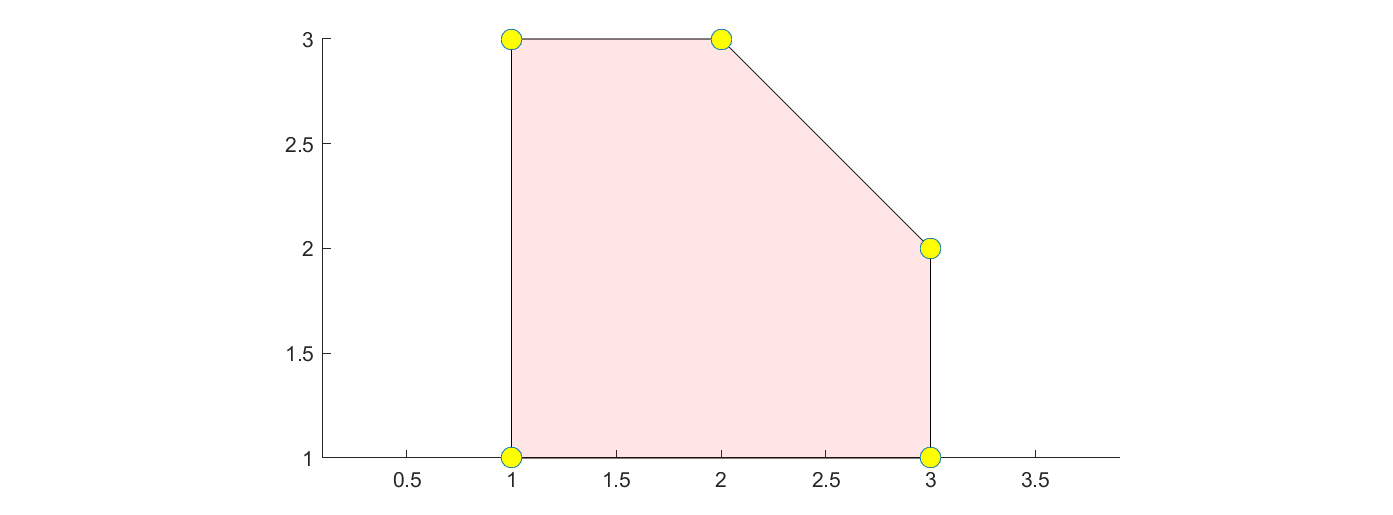# Worst-case norms of matrices

Tags:

Updated:

The robust optimization framework was improved significantly (graph models supported) right after this post was written and simplifies the code below significantly thus making it close to obsolete in practice

I was asked by a colleague today on how to compute the worst-case $$\infty$$-norm of a matrix $$A(p)$$ linearly parameterized in an uncertainty $$p$$ constrained to a polytope.

No simple way came to mind, and using a result by Mangasarian and Shiau 1986 that maximizing the norm of a vector over a polytope is NP-complete for most cases, I guess there should not be any.

Nevertheless, let us write some YALMIP code to compute it, and then generalize the problem formulation. We first note that $$\infty$$-norm of a matrix corresponds to the largest 1-norm of the rows. Hence, all we have to do is to loop over all rows, and compute the worst-case 1-norm of each row.

Unfortunately, worst-case 1-norm is one of the hard cases in Mangasarian 1986. Even worse from our perspective, due to this intractability, adressing uncertain constraints represented using a graph representations and the robust optimization framework of YALMIP is not supported.

For this particular application, the matrix dimension is reasonably small, so we explicitly generate the linear programming formulation of the 1-norm by looking at all combinations of positive and negative terms in the absolute values, i.e. we write $$\left\lvert x(p)\right\rvert +\left\lvert y(p)\right\rvert \leq t$$ as $$x(p)+y(p)\leq t$$, $$-x(p)+y(p) \leq t$$, $$x(p)-y(p)\leq t$$ and $$-x(p)-y(p)\leq t$$. Once we have all these constraints for all rows, we use YALMIPs robust optimization framework to compute the worst-case $$t$$ over the uncertain parameter $$p$$.

To begin with, define a linearly parametrized matrix

n = 4;
A0 = randn(n);
A1 = randn(n);
A2 = randn(n);
p = sdpvar(2,1);
A = A0 + A1*p(1) + A2*p(2);


Define a matrix of size $$2^n \times n$$ containing all combinations of summing up the elements in a row using $$+1$$ and $$-1$$.

combs = [];
for i = 0:2^n-1
combs = [combs;-1+2*dec2decbin(i,n)];
end


Set up all upper bound constraints for the explicit construction of the 1-norm for all rows.

Constraints = [];
t = sdpvar(1);
for row = 1:n
a = A(row,:);
Constraints = [Constraints,combs*a' <= t];
end


Define some cool uncertainty structure

P = [uncertain(p), 1 <= p <= 3, sum(p) <= 5];


Finally minimize the worst-case upper bound

optimize([P, Constraints],t)


The way YALMIP eliminates the uncertainty depends on the structure of the uncertainty set. For the polytopic model above, enumeration will be used, which might be problematic if you have many uncertain variables. In this case it is of no concern though as the uncertainty polytope only has 5 vertices

clf
plot([1 <= p <= 3, sum(p) <= 5]);
v = extreme([1 <= p <= 3, sum(p) <= 5]);
hold on
m = plot(v(1,:),v(2,:),'o');As an alternative though, we can use the duality filter

ops = sdpsettings('robust.lplp','duality');
optimize([uncertain(p),P,Constraints],t,ops)


Note that more advanced uncertainty descriptions can be used. For instance, the following ball-constrained uncertainty is supported and yields a second-order cone program

P = [uncertain(p), p'*p <= 1];
optimize([P, Constraints],t)


Finally, let us find a shift of the eigenvalues of A, such that the worst-case infinity norm is minimized.

s = sdpvar(1);
A = A + s*eye(n);
Constraints = [];
for row = 1:n
a = A(row,:);
Constraints = [Constraints,combs*a' <= t];
end
P = [uncertain(p), 1 <= p <= 3, sum(p) <= 5];

optimize([P, Constraints],t)